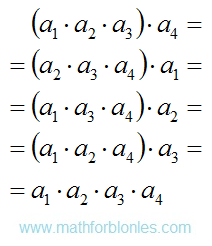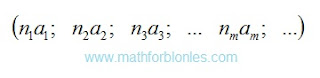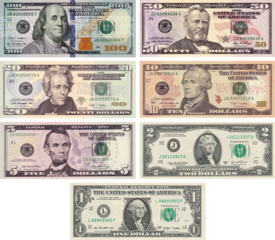## 8.26.2019

### The formation of the set

I have already told you that set theory is a herd theory by which shamans try to sort out "sea urchins" of reality. How do they do that? How does the formation of the set actually occur?

Initially, individual elements stand out from reality. After that, a set are formed from some elements that have a common property. Shamans always know in advance how a set they want to show us.

I will show the process by example. We select "red solid with holes" - these are our elements. At the same time, we see that these elements are with a bow, and there are without a bow. After that, we select some of the elements and form a set of "with a bow." This is how shamans get their food, tying their set theory to reality.

Now let's take a “solid with holes with a bow” and combine these elements by color, selecting red ones. We got a set of red. Now the question is: the resulting sets "with a bow" and "red" - is it the same set or two different sets? Only shamans know the answer.

This simple example shows that set theory is completely useless when it comes to reality. What's the secret? We formed a set of "red solid with holes with a bow". The formation took place in four different units of measurement: color (red), strength (solid), integrity (with holes), jewelry (with a bow). Only a set of units of measurement allows us to adequately describe real objects in the language of mathematics. Here is how it looks.The formation of the set

The letter "a" with different indices indicates different units of measurement. In brackets, the units of measurement that are considered at the preliminary stage are highlighted. The unit of measure by which the set is formed is the set out of brackets. The last line shows the final result - an element of the set. If we use units of measure to form a set, then the result does not depend on the order of our actions. And this is mathematics, and not the dancing of shamans with tambourines. Shamans can "intuitively" reach the same result, arguing for its "evidence", because units of measurement are not included in their "scientific" arsenal.

Using units of measure, it is very easy to split one set into several subsets, or to combine several sets into one set. Let's take a closer look at the algebra of this process.

## 8.10.2019

### Element of the set

If mathematicians cannot reduce the concept of "set" to other concepts, then they do not understand anything in mathematics. I answer the question: how are the elements of one set different from the elements of another set? The answer is very simple: numbers and units of measurements.

Today everything belongs to some kind of set (as mathematicians assure us). By the way, did you look at yourself in the mirror? Have you seen on your forehead a list of those sets to which you belong? And I have not seen such a list. I will say more - not a single thing in reality has a tag with a list of sets to which this thing belongs. Sets are shamans' inventions. How do they do it? Let's recall the history and see how the elements of the set looked like before the mathematician-shamans pulled them into their sets.

Once upon a time, when no one had heard of mathematics, and only trees and Saturn had rings, huge herds of wild elements of sets roamed the physical fields (after all, shamans had not yet invented mathematical fields). They looked something like this.Sea urhin

Yes, do not be surprised, from the point of view of mathematics, all the elements of sets are most similar to sea urchins - from one point, like needles, units of measurements stick out in all directions. For those who missed something, I remind you that any unit of measure can be represented geometrically as a segment of arbitrary length, and a number as a point. Geometrically, any value can be represented as a bunch of segments sticking out in different directions from one point. This point is point zero. I will not draw this work of geometric art (no inspiration), but you can easily imagine it.

What units of measurements form an element of the set? Any, describing this element from different points of view. These are the ancient units of measurement used by our ancestors and which everyone has long forgotten about. These are the modern units of measurement that we use now. These are units of measurement unknown to us that our descendants will come up with and which they will use to describe reality.

We figured out the geometry - the proposed model of the elements of the set has a clear geometric representation. What about physics? Units of measurement - this is the direct connection of mathematics with physics. If shamans do not recognize units of measurement as a full-fledged element of mathematical theories, this is their problem. Personally, I can’t imagine real math science without units of measurement. That is why, at the very beginning of the story about set theory, I spoke of it as a Stone Age.

But let us pass to the most interesting thing - to the algebra of elements of sets. Algebraically, any element of a set is a collection of different quantities. It looks like this.Element of the set

I deliberately do not apply the conventions adopted in set theory, since we consider the element of the set in its natural environment before the advent of set theory. Each pair of letters designates a separate quantity consisting of a number indicated by the letter "n" and a unit of measurement indicated by the letter "a". Indexes indicate that the numbers and units are different. One element of the set can consist of an infinite number of quantities (how much imagination we have and our descendants). Each value is geometrically depicted as a separate segment. In the example with the sea urchin, one value is one needle.

How do shamans form sets of different elements? In fact, by units of measurement or by numbers. Without understanding anything in mathematics, they take different sea urchins and carefully examine them in search of the only needle by which they form a multitude. If there is such a needle, then this element belongs to the set, if there is no such needle, it is an element of another set. Shamans tell us tales about thought processes and a single whole.

As you may have guessed, one and the same element can belong to very different sets. Further I will show you how the sets, subsets and other attributes of shamans are formed.

## 8.09.2019

### Set and multiset

The concepts of “set” and “multiset” are two trump ace in the sleeves of a sharpie, one in each sleeve. The sharpie takes out from the sleeve that trump ace, which is more convenient for him to get. In any case, the sharpie always wins. In the same way, any mathematician will always justify set theory.

There can’t be two identical elements in a set, but if there are identical elements in a set, such a set is called a “multiset.” Intelligent beings can never understand such a logic of absurdity. This is the level of talking parrots and trained monkeys. Mathematicians act as ordinary animal trainer, preaching to us their absurd ideas.

Once the engineers who built the bridge, during the testing of the bridge, were in a boat under the bridge. If the bridge collapsed, a mediocre engineer died under the rubble of his creation. If the bridge could withstand the load, a talented engineer built other bridges.

No matter how mathematicians hide behind the phrase “mathematics studies abstract concepts”, there is one umbilical cord that inextricably connects them with reality. This umbilical cord is money. We apply the mathematical set theory to the mathematicians themselves.

We studied mathematics very well and now we are giving out salaries. Here comes a mathematician for his money. We count the whole amount to him and lay out on his table on different piles, into which we place banknotes of the same denomination. Then we take one banknote from each pile and hand over to the mathematician his "mathematical set of salary." We explain the mathematics that he will receive the remaining bills only when he proves that a set without the same elements is not equal to a set with the same elements. After that, the most interesting will happen.Set and multiset

First of all, the logic of the deputies will work: "It can be applied to others, but not to me!" Further, the mathematician will assure us that on banknotes of the same denomination there are different numbers of banknotes, therefore they cannot be considered the same elements of the set. Well, we count the salary in coins - there are no numbers on the coins. Here, the mathematician will frantically recall physics: different coins have different amounts of dirt, the crystal structure and arrangement of atoms of each coin is unique ...

And now I have the most interesting question: where is the line behind which the elements of the multiset turn into the elements of the set and vice versa? Such a facet does not exist - shamans decide everything, science is absent here.

You see that the same set of elements is both a multitude and a multiset at the same time. How right? And here the mathematician-shaman-schuller takes an ace from his sleeve and begins to tell us either about the multitude or the multiset. In any case, he will convince us that the theory of sets is correct.

To understand how modern shamans apply set theory to reality, it is enough to answer one question: how do the elements of one set differ from the elements of another set? I will show you how to do it.

### Set theory

Set theory is the stone age of mathematics. Only shamans know how to properly form the set. Let's look at set theory from the side and reveal some of the secrets of shamans. To understand the essence of set theory, it is necessary to consider another mathematical concept - a function. The function sets the correspondence between the elements of two sets. Each element from the herd of hunters is associated with one or more elements from the herd of prey, each element from the herd of prey is assigned one or more elements from the herd of hunters. Only shamans know what kind of herd belongs to and how to divide prey properly.

What is set theory? This is a herd theory. What will happen if we combine a herd (set) mathematicians and a herd (set) sheep: sheeps with a mathematical education or mathematicians with sheep’s brains? I don’t know what set theory says about the results of such a union, but in reality mathematicians will have a great barbecue picnic. I have nothing against set theory, as one of the mathematical tools. But to stand up for “scientific” theories based on such a primitive and vague concept is already too much.

Any theory should be tested by practice, even mathematical. I will show you an example of the practical application of set theory by the example of such concepts as “set” and “multiset”.

## 1.12.2019

### Achilles and the tortoiseAchilles and the tortoise

In the fifth century BC, the ancient Greek philosopher Zeno of Elea formulated his famous aporia, the most famous of which is the Achilles and the Tortoise aporia. Here’s how it sounds:

Suppose Achilles runs ten times faster than a turtle, and is a thousand paces behind it. During the time that Achilles has run this distance, the tortoise will crawl a hundred steps in the same direction. When Achilles runs a hundred paces, the tortoise crawls ten more steps, and so on. The process will continue indefinitely, Achilles will never catch up with the tortoise.

Another version of the aporia "Achilles and the tortoise":

In a race, the quickest runner can never overtake the slowest, since the pursuer must first reach the point whence the pursued started, so that the slower must always hold a lead.

This reasoning was a logical shock for all subsequent generations. Aristotle, Diogenes, Kant, Hegel, Hilbert ... All of them in one way or another viewed the aporia of Zeno. The shock turned out to be so strong that discussions are continuing at the present time; the scientific community has not yet succeeded in reaching a general opinion on the essence of paradoxes. Mathematical analysis, set theory, new physical and philosophical approaches were involved in the study of the question; none of them has become a generally accepted solution. Everyone understands that this is a hoax, but no one understands what it is.

From the point of view of mathematics, Zeno in his aporia clearly demonstrated the transition from quantity to an inverse quantity. This transition implies the use of variable units of measurement instead of constants. As far as I understand, the mathematical apparatus for using variable units of measurement has either not yet been developed or has not been applied to the aporia of Zeno. The application of our usual logic leads us into a trap. We, by the inertia of thinking, apply constant units of time to the reciprocal. From a physical point of view, it looks like a slowing down of time to its full stop at the moment when Achilles approaches the turtle. If time stops, Achilles can no longer overtake the turtle.

If we turn the logic we are used to, everything falls into place. Achilles runs at a constant speed. Each subsequent segment of its path is ten times shorter than the previous one. Accordingly, the time spent on its overcoming is ten times less than the previous one. If you use the concept of "infinity" in this situation, then it will be correct to say "Achilles will catch up to the tortoise infinitely quickly".

How to avoid this logical trap? Stay in constant time units and don’t go to inverse quantity. In the language of Zeno, it looks like this:

In the time that Achilles has run a thousand steps, the tortoise will crawl a hundred steps in the same direction. Over the next interval of time equal to the first, Achilles will run a thousand more steps, and the turtle will crawl a hundred steps. Now Achilles is eight hundred paces ahead of the tortoise.

This approach adequately describes reality without any logical paradoxes. But this is not a complete solution. On the aporia of Zeno "Achilles and the Turtle" is very similar to Einstein's statement about the irresistible speed of light. We still have to study, rethink and solve this problem. And the solution must be sought not in infinitely large numbers, but in units of measurement.

Another interesting aporia of Zeno tells of a flying arrow:

A flying arrow is motionless, because at each moment of time it is motionless, and since it is motionless at each moment of time, it is always motionless.

In this aporia, the logical paradox is overcome very simply - it is enough to clarify that at each moment in time a flying arrow is stationary at different points in space, which, in fact, is movement.

## 2.10.2018

### Trapezoid

In Wikipedia there is a page of a trapezoid. In the drawing it is shown that any trapezoid can be turned into a rectangle.Trapezoid
Let's look at algebra. These are formulas for calculation of lengths of diagonals of a trapezoid.Diagonals of trapezoid
We substitute in these formulas data for a rectangle.Diagonals of rectangle
If to trust these formulas, the rectangle has no diagonals. Even schoolboys or schoolgirls can make what mathematicians couldn't make – to execute verification of the solution of a task. It is the actual level of modern mathematics – any statement of mathematicians can be false.

Height of a trapezoid

Height of a trapezoid is determined by the area of a triangle. The area of a triangle is calculated on Heron's formula. The sizes of the parties of a trapezoid allow to receive a triangle which has the same height as a trapezjid. The cunning trick of mathematicians allows to calculate length of diagonals of a trapezoid.Height of a trapezoid
When the legs of a trapezoid are parallel, the triangle disappears and the cunning trick ceases to work. If to determine height of a trapezoid by the area of a trapezoid, then no problems arise upon transition to a rectangle or a parallelogram.Height of a rectangle
Conclusion: cunning tricks of mathematicians can result in false results.

## 3.02.2017

### Inverse transformations

Last time we transformed the law of cosines and a Pythagorean theorem to the sum of a line segments. Now we will execute inverse transformations.Inverse transformations

In inverse transformations I made everything very simply. The minus sign from a formula disappeared. The problem is that we are not able to measure angles correctly. Than differ a angle 0 degrees from a angle of 180 degrees?Measurement of a angle
It is possible to assume that if a line segment one, then a angle is equal 0 degrees. If a line segments two, then a angle is equal 180 degrees.

The transformations executed by us show that the mathematics has no separate areas of mathematics: "arithmetic", "algebra", "geometry" or "trigonometry". The mathematics is a single whole.

The mathematics is DNA of the nature. Further we will continue to study a cosine gene in a cosine law.

If you liked the publication and you want to know more, help me with working on by other publications.